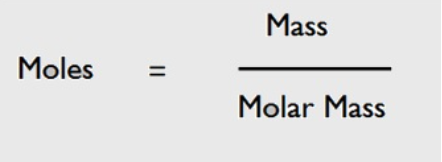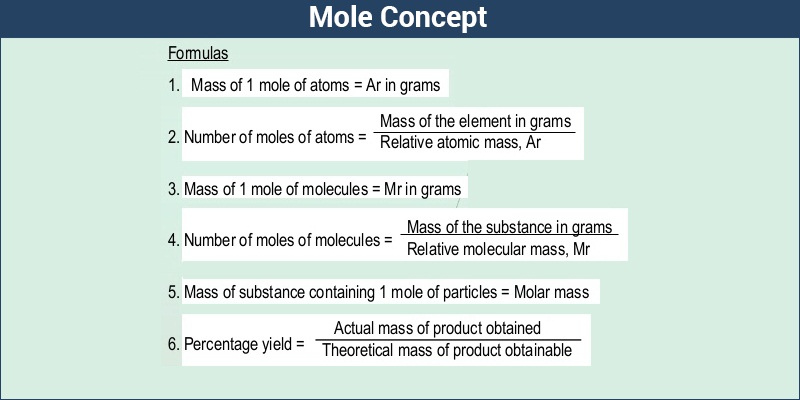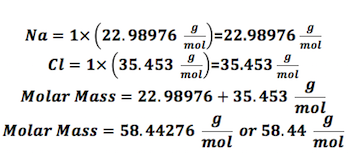# Molar Mass Study Guide

INTRODUCTION

Have you ever wondered what the exact number of molecules present in a football you are playing with or the water you are drinking is? We know by now that atoms or molecules are the smallest units when considering both mass and size. The weight of one sample mole is the molar mass. The molar mass is calculated by adding all the atomic masses of each and every atom in a molecule. How can molar mass be defined then?

### WHAT IS MOLAR MASS IN CHEMISTRY?

The molar mass of any substance is defined as the smallest mass unit of that compound with one-twelfth of the mass of one carbon that would be 12 atoms. In any substance, the number of entities present can always be defined as a mole.### WHAT IS THE MOLAR MASS UNIT?

You can take the standard unit to be g mol⁻¹ and the SI one as kg mol⁻¹. The number of atoms that is observed in 12g of 12C isotope is the number of particles you would also observe in 1 mole of the substance. Remember, no matter the substance, the mole of any substance would always contain the same entity number.

A spectrometer was used to measure the mass of carbon-12 atoms, and it was found to be 1.992648x 10⁻³g. The number of entities in one mole is crucial while calculating the mass in chemistry and is known as the Avogadro number, which is 6.02214076 x 10²³.

#### Periodic Table and Molar Mass

As we already know, the molar mass is the mass in g of 1 mole that is 6.02 x 1023 particles. Therefore, in the majority of cases, you just need the atomic weight of an element on the periodic table to find out the molar mass.

To understand this better, you will find the atomic weight written under the element name and element symbol.

For instance, if you look at carbon, its atomic weight is 12.01. Therefore its molar mass is 12.01 gm/mole.

However, there are some exceptions. This exception is tagged to the elements which are present in different forms rather than 1 unbonded atom. There are 7 elements in the periodic tale that are diatomic. These elements include H, O, N, I, Br, F, and, CL. These are the special ones, here you have to multiply the atomic weight by 2.

Further, there are some elements that have more than 2 atoms. For instance, Phosphorus has 4 atoms, sulphur has 8 atoms, and selenium also has 8 atoms.

### MASS AND MOLAR MASS FORMULA

It might seem easy to calculate the formula masses of molecules, atoms, and other such compounds; however, finding individual weights of these particles does not serve any useful purpose. They should be expressed at a more macroscopic level, and the bridge between this particulate and the macroscopic level is considered as the molar mass.

``                   Molar mass = mass/mole = g/mol``### Calculating Molar Mass from Atomic Masses

Molar Mass – Mass of 1 mole of the given substance.

You can calculate the molar mass of any compound by summing up the atomic weights of the present atoms.

For instance: let us calculate the Molar mass of H2O

Molar mass of H2O = 2(Atomic weight of hydrogen) + 1(Atomic weight of oxygen) = 2 x 1 + 1 x 16 = 18 grams/mole

### HOW CAN YOU EASILY FIND THE MOLAR MASS FOR DIFFERENT COMPOUNDS?

Let us take an example to understand how to calculate the molar mass of NaCl.• Using the periodic table, find the atomic masses: For each element, you must figure out the atomic mass using the periodic table. For any element, the number that appears on the top left represents atomic mass. The atomic mass of sodium would be 22.98976 g/mol, and that of chlorine would be 35.453 g/mol.
• Count the number of atoms present in each element: Since there are no subscripts, that means there is only one sodium and one chlorine atom in this compound.
• Now Find the molar mass: Now that you know the atomic mass and how many atoms of the compound are involved, you can find the molar mass as shown in the example.

## CONCLUSION

• Molar mass can be defined as the smallest unit of mass of any compound and is the twelfth of one carbon mass – 12 atoms.
• The standard unit is g mol⁻¹ and the SI one as kg mol⁻¹.
• You can find the molar mass if you know the atomic mass and number of atoms involved in each element.

### FAQs

1. Is mass equal to molar mass?

Molar mass is considered to be the same as the formula mass.

2. What is meant by molar mass?

Molar mass can be defined as the smallest unit of mass of any compound and is the twelfth of one carbon mass- 12 atoms.

We hope you enjoyed studying this lesson and learned something cool about Molar Mass! Join our Discord community to get any questions you may have answered and to engage with other students just like you! Don’t forget to download our App to experience our fun VR classrooms – we promise, it makes studying much more fun! 😎

]]>